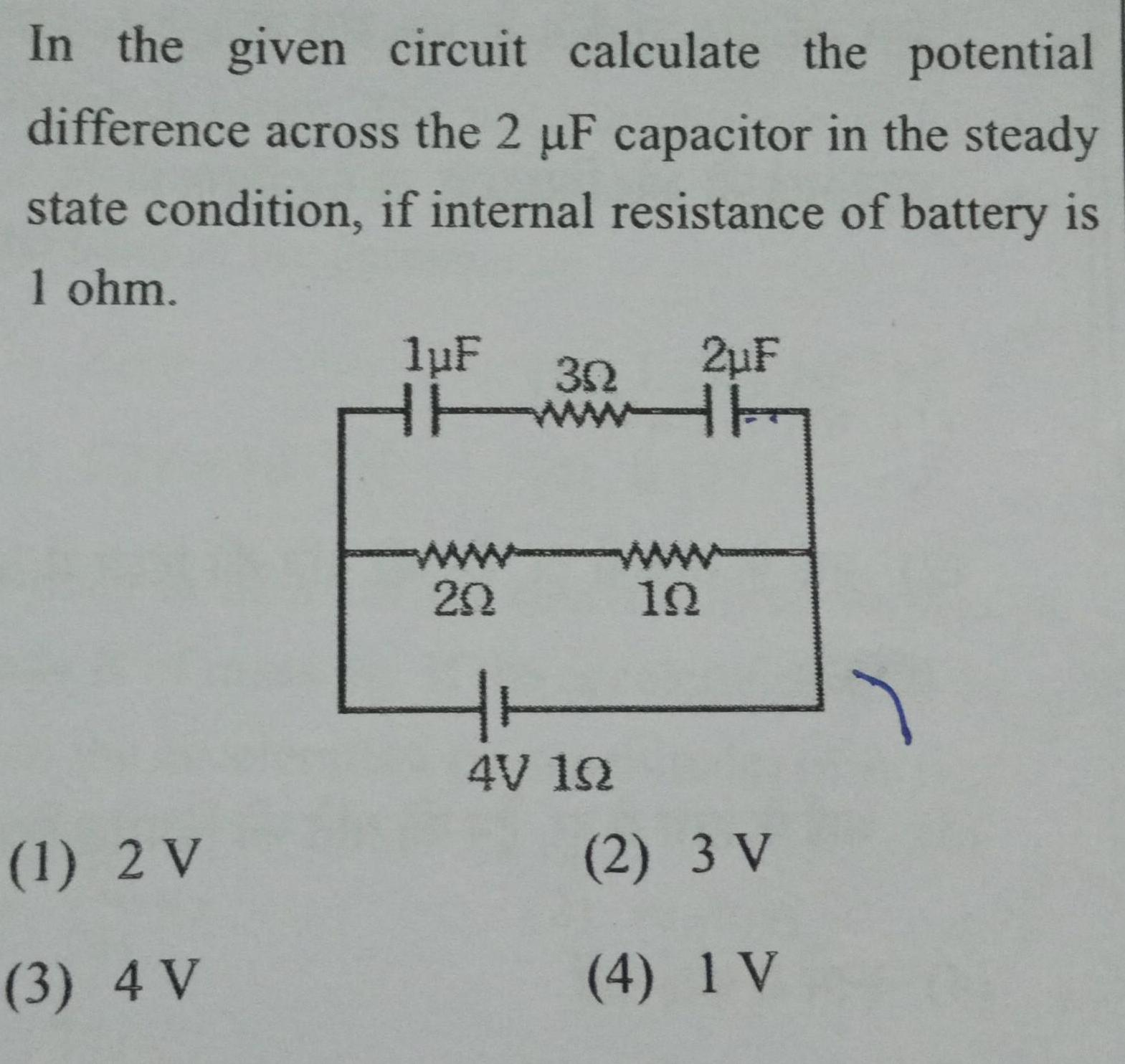Physics
Capacitors
In the given circuit calculate the potential difference across the 2 F capacitor in the steady state condition if internal resistance of battery is 1 ohm 1 2 V 3 4 V 1 F w 202 32 4V 12 2 F 192 2 3 V 4 1V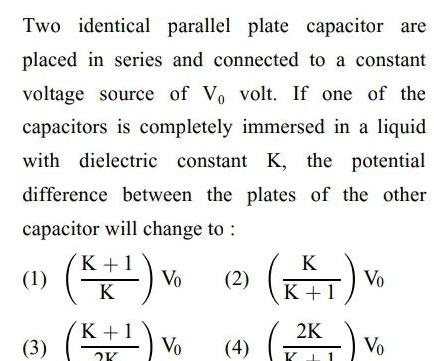Physics
Capacitors
Two identical parallel plate capacitor are placed in series and connected to a constant voltage source of Vo volt If one of the capacitors is completely immersed in a liquid with dielectric constant K the potential difference between the plates of the other capacitor will change to Vo 2 K 1 K 3 K 1 1 Vo 4 K K 1 2K K Vo Vo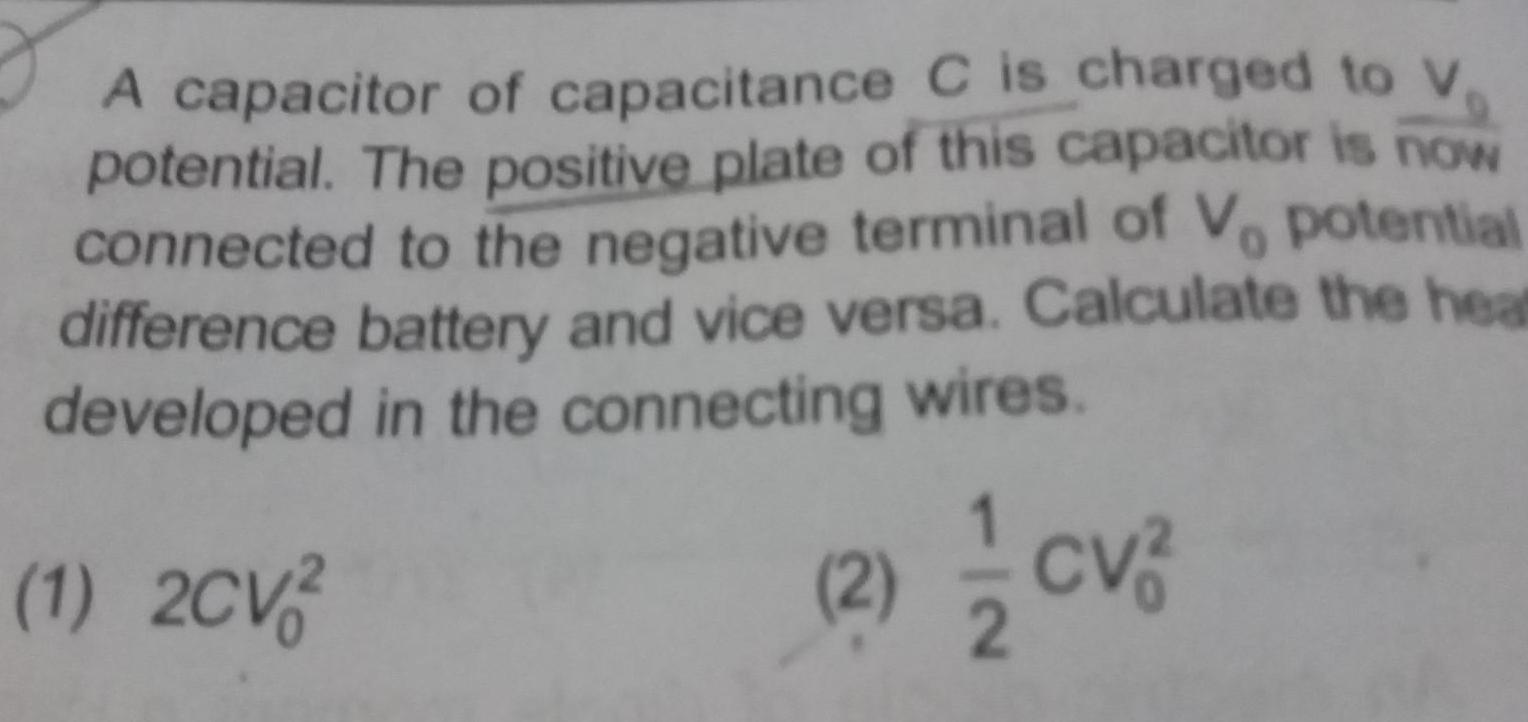Physics
Capacitors
A capacitor of capacitance C is charged to V D potential The positive plate of this capacitor is now connected to the negative terminal of Vo potential difference battery and vice versa Calculate the heat developed in the connecting wires 1 2CV2 2 CV 2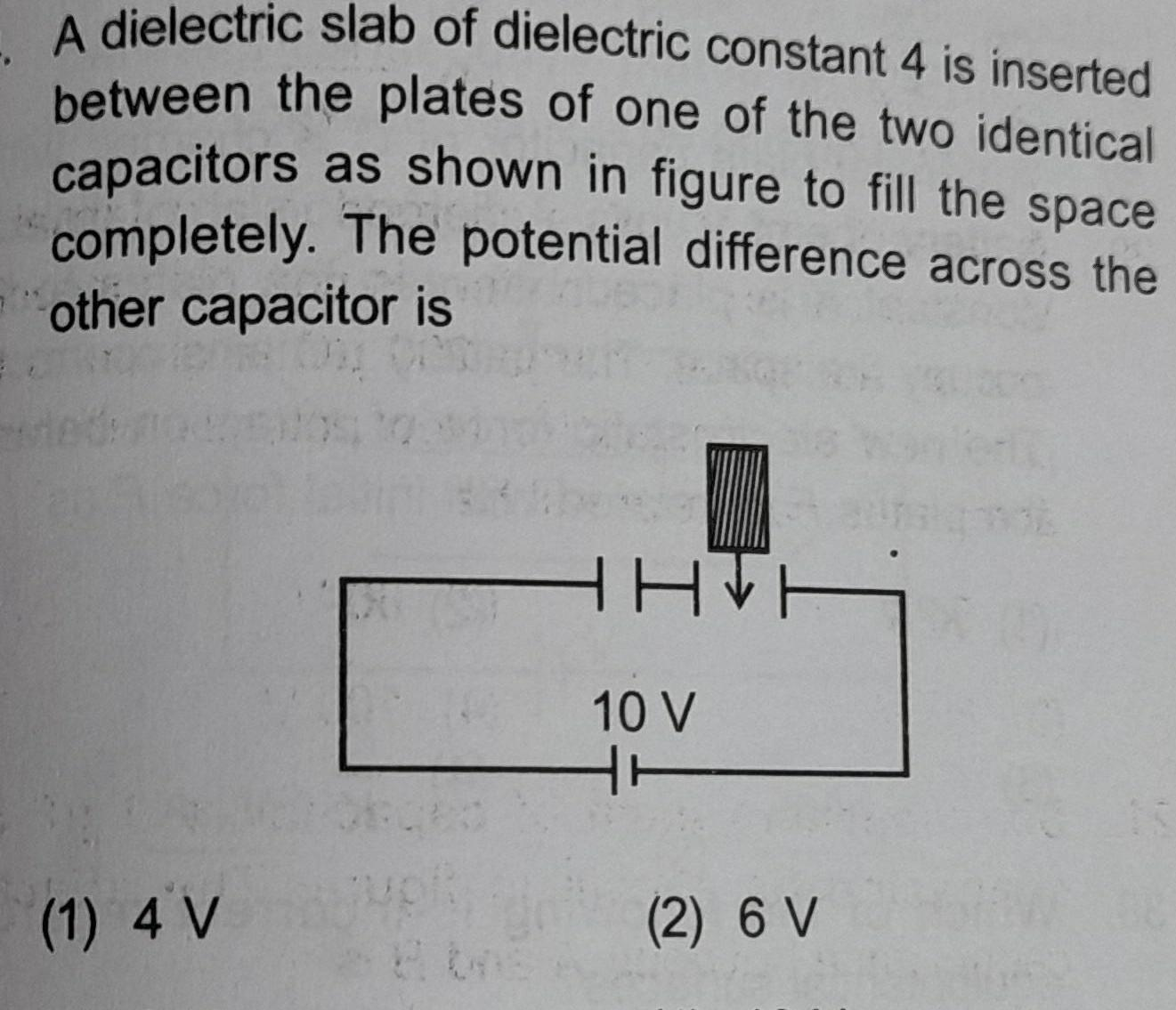Physics
Capacitors
A dielectric slab of dielectric constant 4 is inserted between the plates of one of the two identical capacitors as shown in figure to fill the space completely The potential difference across the other capacitor is 1 4 V HHH 10 V 1 2 6 V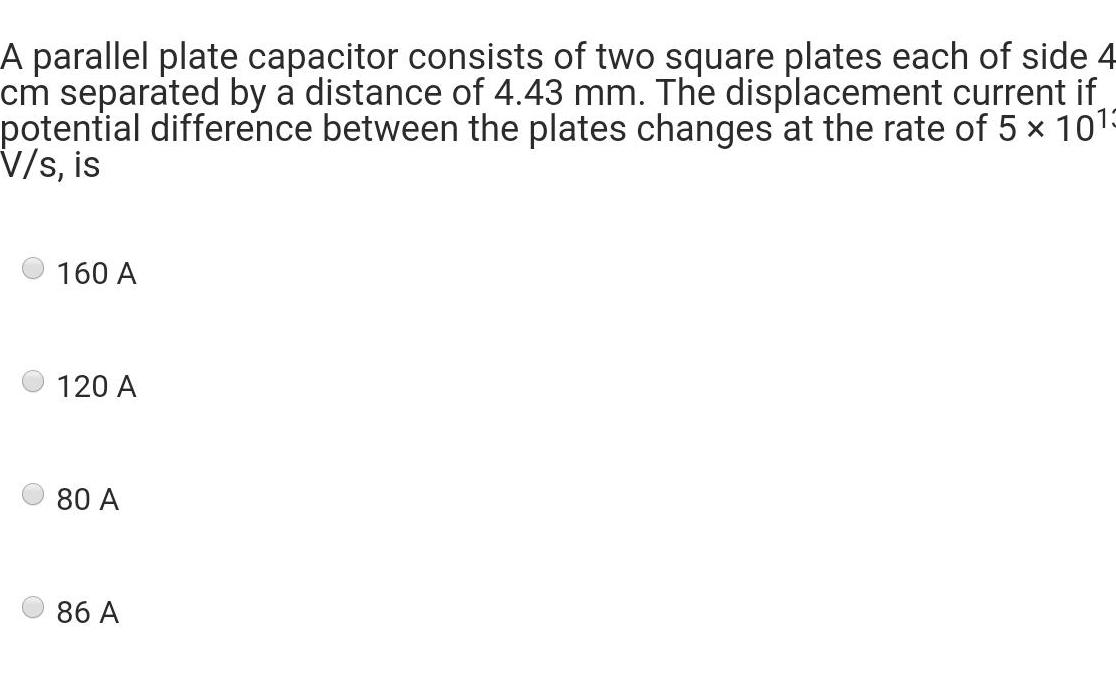Physics
Capacitors
A parallel plate capacitor consists of two square plates each of side 4 cm separated by a distance of 4 43 mm The displacement current if potential difference between the plates changes at the rate of 5 x 10 V s is 160 A 120 A 80 A 86 A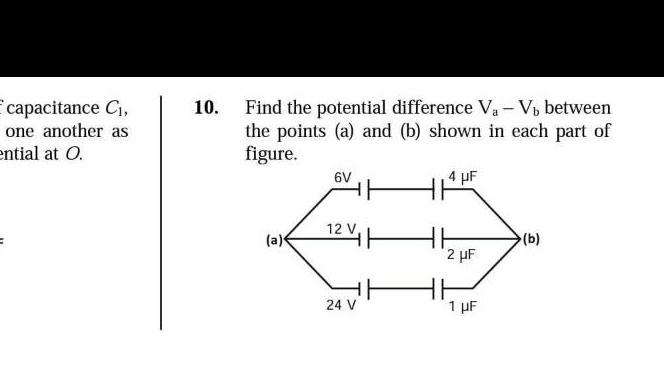Physics
Capacitors
capacitance C one another as ential at O 10 Find the potential difference V V between the points a and b shown in each part of figure a 6V 24 V 4 F 2 F 1 F b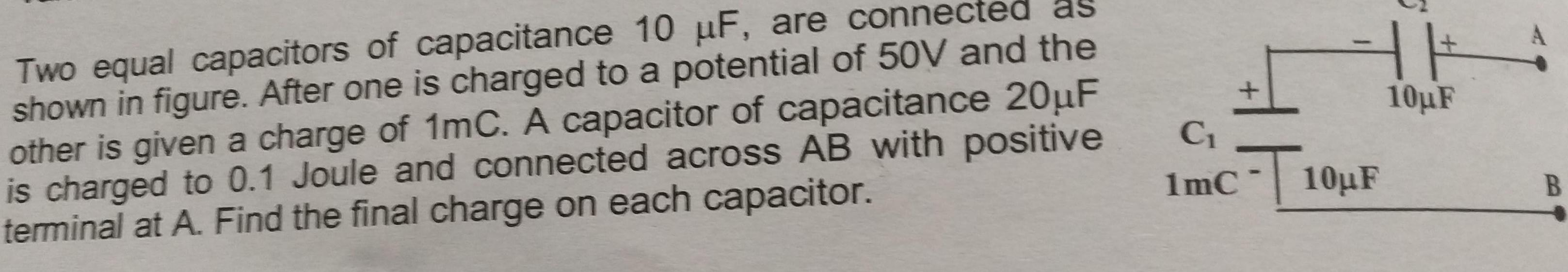Physics
Capacitors
Two equal capacitors of capacitance 10 F are connected as shown in figure After one is charged to a potential of 50V and the other is given a charge of 1mC A capacitor of capacitance 20 F is charged to 0 1 Joule and connected across AB with positive terminal at A Find the final charge on each capacitor HE 10 F C Imc T T10 F B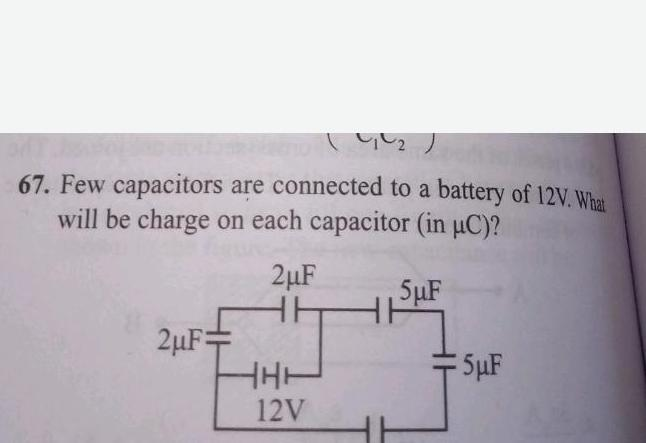Physics
Capacitors
67 Few capacitors are connected to a battery of 12V What will be charge on each capacitor in C 5 F 2 F 2 F HH HHH 12V 5 F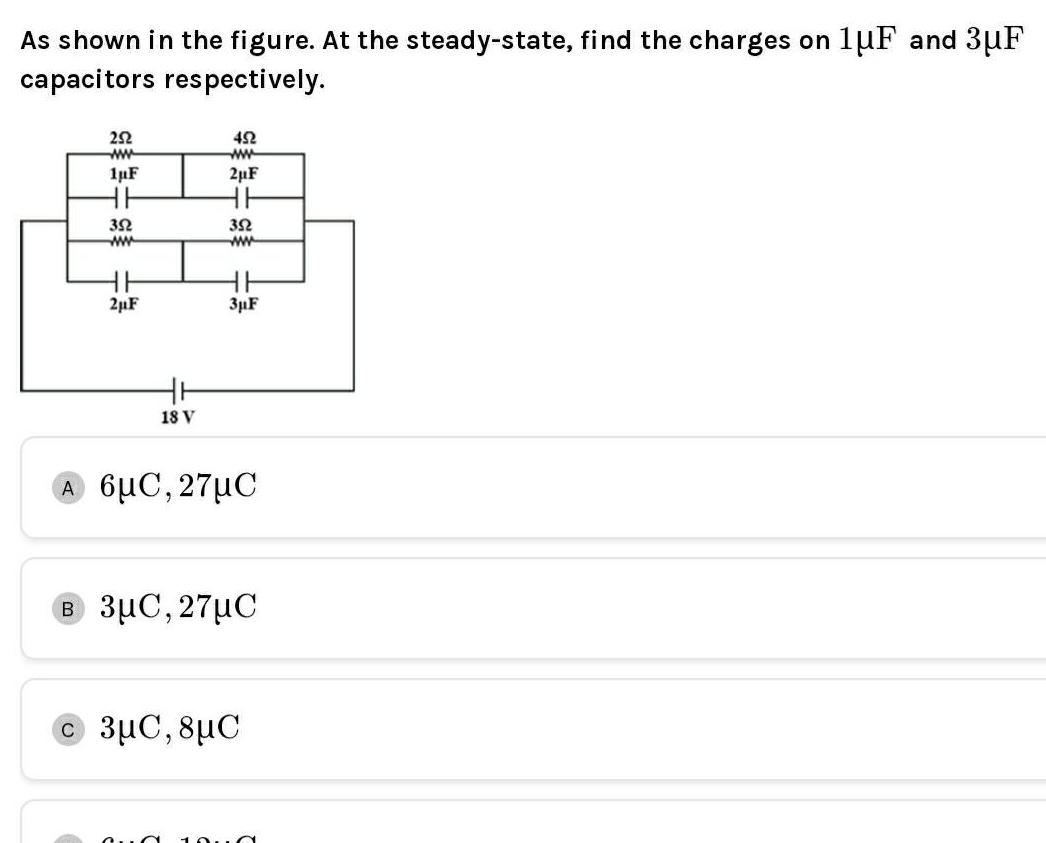Physics
Capacitors
As shown in the figure At the steady state find the charges on 1uF and 3 F capacitors respectively 252 ww 1uF HH 352 ww 2 F 18 V 452 ww 2 F HH 352 www 3 F A 6 27 C 27 C 3 C 8 C a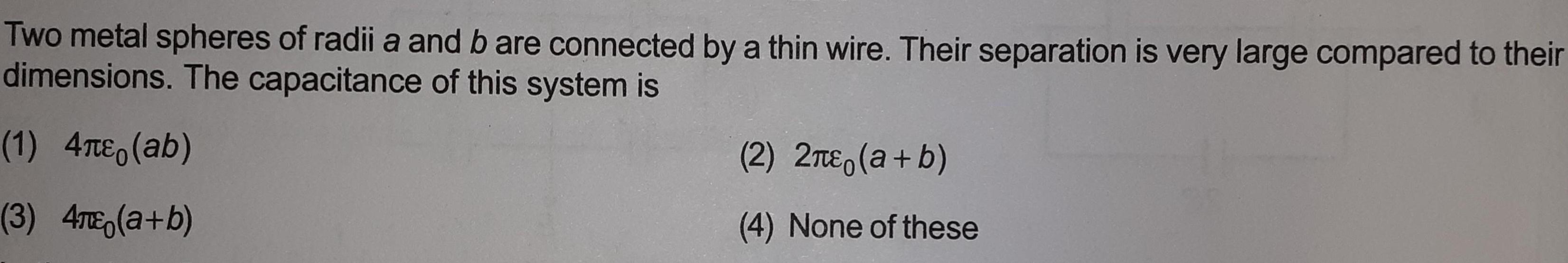Physics
Capacitors
Two metal spheres of radii a and b are connected by a thin wire Their separation is very large compared to their dimensions The capacitance of this system is 1 4 ab 3 4TE a b 2 2 a b 4 None of these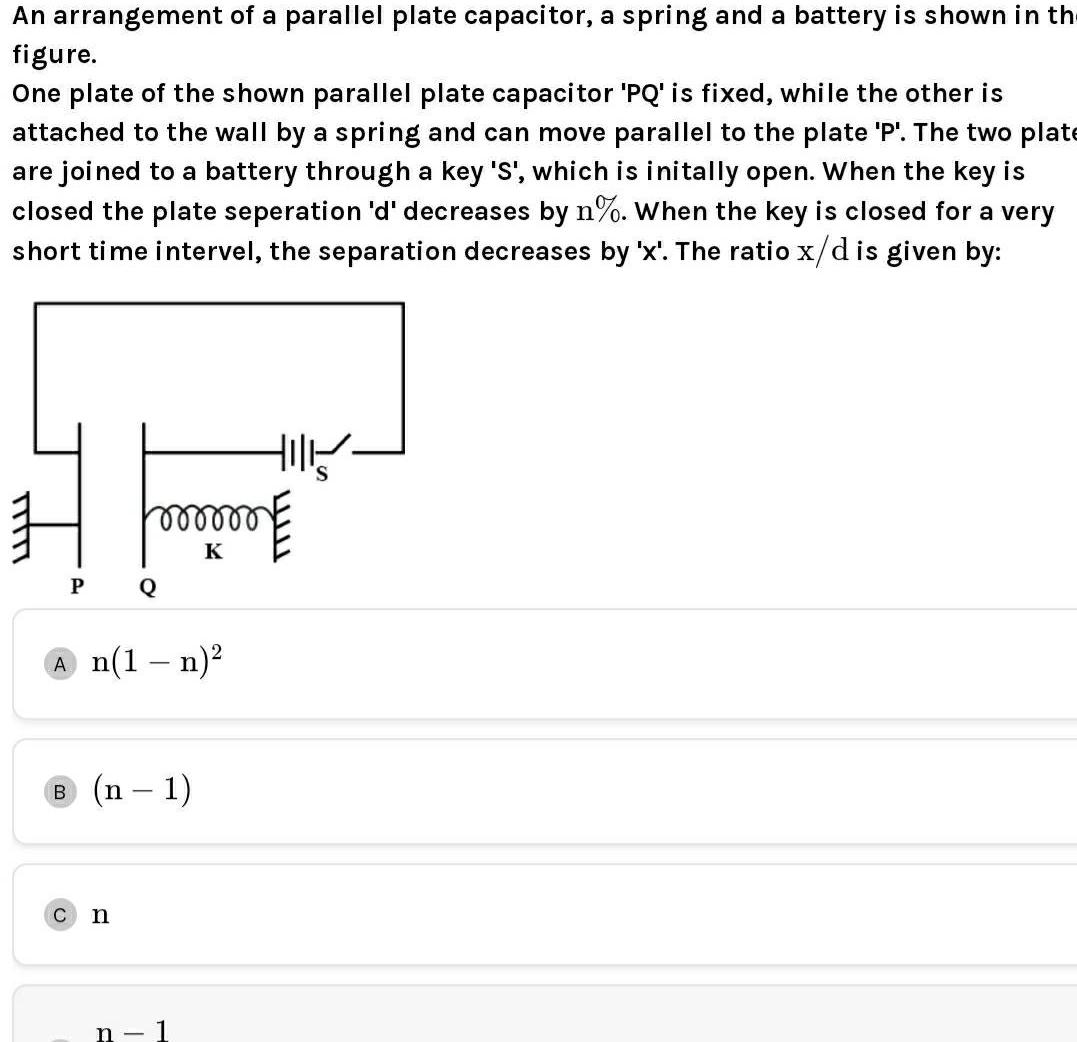Physics
Capacitors
An arrangement of a parallel plate capacitor a spring and a battery is shown in the figure One plate of the shown parallel plate capacitor PQ is fixed while the other is attached to the wall by a spring and can move parallel to the plate P The two plate are joined to a battery through a key S which is initally open When the key is closed the plate seperation d decreases by n When the key is closed for a very short time intervel the separation decreases by x The ratio x d is given by H P Hill mmmm me K Q A n 1 n C n B n n 1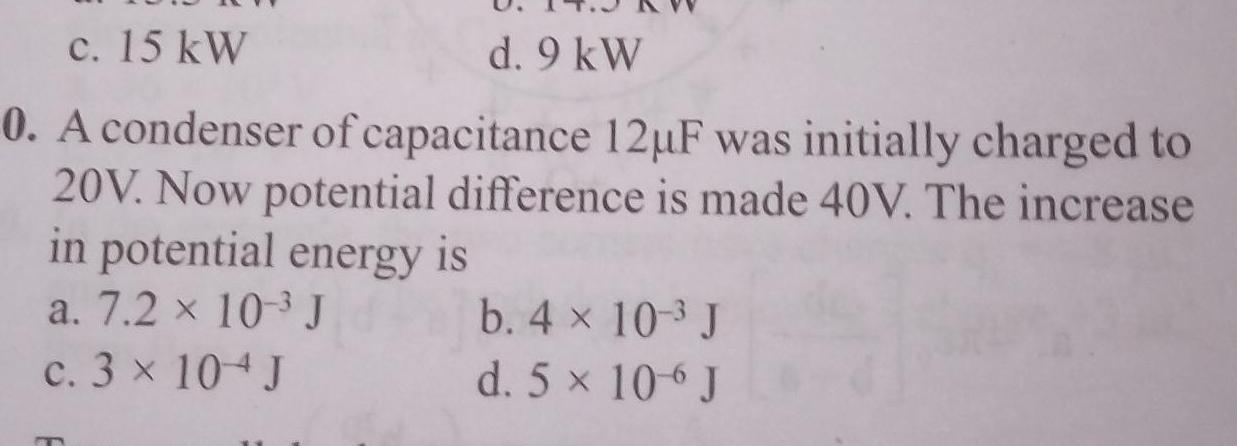Physics
Capacitors
c 15 kW d 9 kW 0 A condenser of capacitance 12 F was initially charged to 20V Now potential difference is made 40V The increase in potential energy is a 7 2 x 10 J c 3 x 104 J m b 4 10 3 J d 5 x 106 J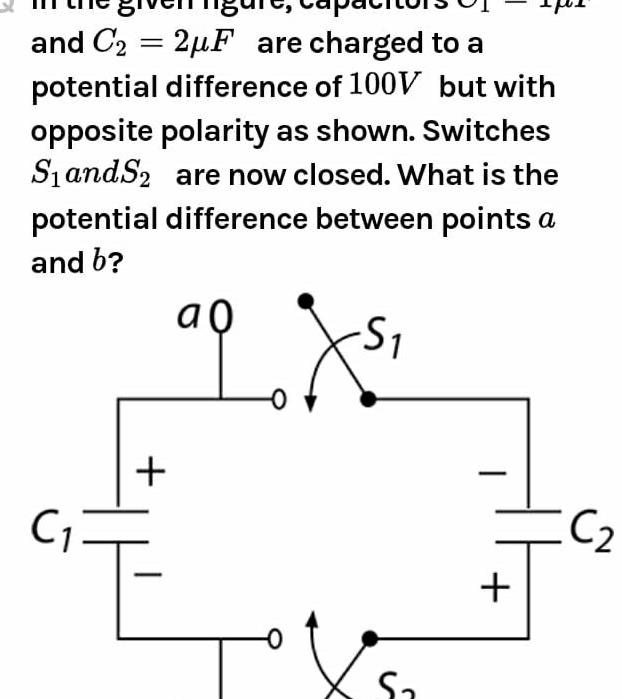Physics
Capacitors
and C 2 F are charged to a potential difference of 100V but with opposite polarity as shown Switches SiandS are now closed What is the potential difference between points a and b ao S C 0 X S C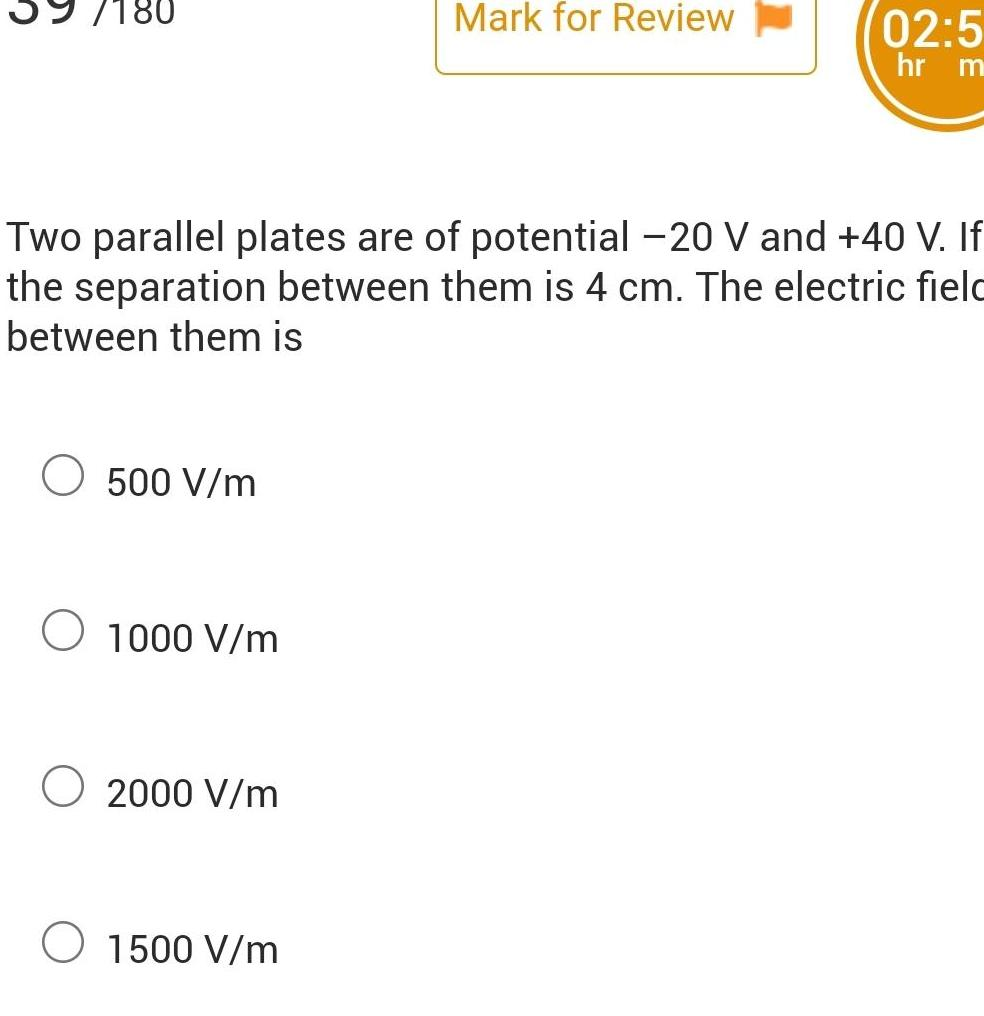Physics
Capacitors
180 O 500 V m Two parallel plates are of potential 20 V and 40 V If the separation between them is 4 cm The electric field between them is O 1000 V m 2000 V m Mark for Review O 1500 V m 02 5 hr m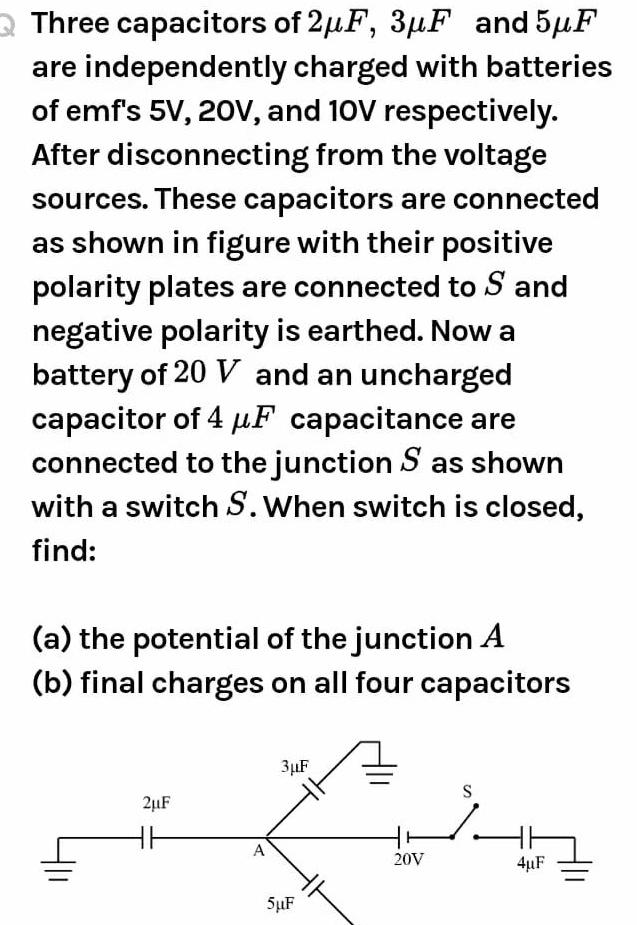Physics
Capacitors
Three capacitors of 2 F 3 F and 5 F are independently charged with batteries of emf s 5V 20V and 10V respectively After disconnecting from the voltage sources These capacitors are connected as shown in figure with their positive polarity plates are connected to S and negative polarity is earthed Now a battery of 20 V and an uncharged capacitor of 4 F capacitance are connected to the junction S as sh with a switch S When switch is closed find a the potential of the junction A b final charges on all four capacitors 2 F HH A 3 F 5 F 20V S 4 F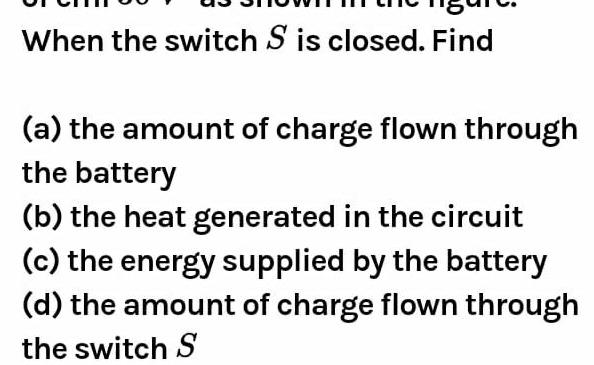Physics
Capacitors
When the switch S is closed Find a the amount of charge flown through the battery b the heat generated in the circuit c the energy supplied by the battery d the amount of charge flown through the switch S